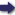(London :  Kegan Paul, Trench, Trübner & Co.,  1910.)

 Tools

## Search this bookPrev Page 313 Next``` CHAPTER XXXI. 313 dian. Hereby people are frequently misled to think that the 4800 yojanas are the corrected circumference for the city of Ujain, If we calculate it (according to Brahmagupta's correction), we find the latitude of Ujain to be i6\ degrees, whilst in reality it is 24 degrees. The author of the canon Karana-tilaka makes this correction in the following way. He multiplies the diameter of the earth by 12 and divides the product by the equinoctial shadow of the place. The gnomon stands in the same relation to this shadow as the radius of the parallel circle of the place to the sine of the lati¬ tude of the place, not to the sinus totus. Evidently the author of this method thinks that we have here the same kind of equation as that which the Hindus call Theequa- • /1/-7- 77 ■ -, 7 • iiowvyasta- vyastatrairasika, i.e. the places with the retrograde motion. traird.iika. An example of it is the following. If the price of a harlot of 15 years be, e.g. 10 denars, how much will it be when she is 40 years old ? The method is this, that you multiply the first number by the second (15 x 10 = 150), and divide the pro¬ duct by the third number (150 : 40 = 3|-). Then the quotient or fourth number is her price when she has become old, viz. 3f denars. Now the author of the ICctr an a-tilaka, after having found that the straight shadow increases with the lati¬ tude, whilst the diameter of the circle decreases, thought, according to the analogy of the just mentioned calcula¬ tion, that between this increase and decrease there is a certain ratio. Therefore he maintains that the diameter of the circle decreases, i.e. becomes gradually smaller than the diameter of the earth, at the same rate as the straight shadow'i?7C7'gases. Thereupon he calculates the corrected circumference from the corrected diameter. After having thus found the longitudinal difference between two places, he observes a lunar eclipse, and fixes in day-minutes the difference between the time of its appearance in the two places. Pulisa multiplies ```Prev Page 313 Next